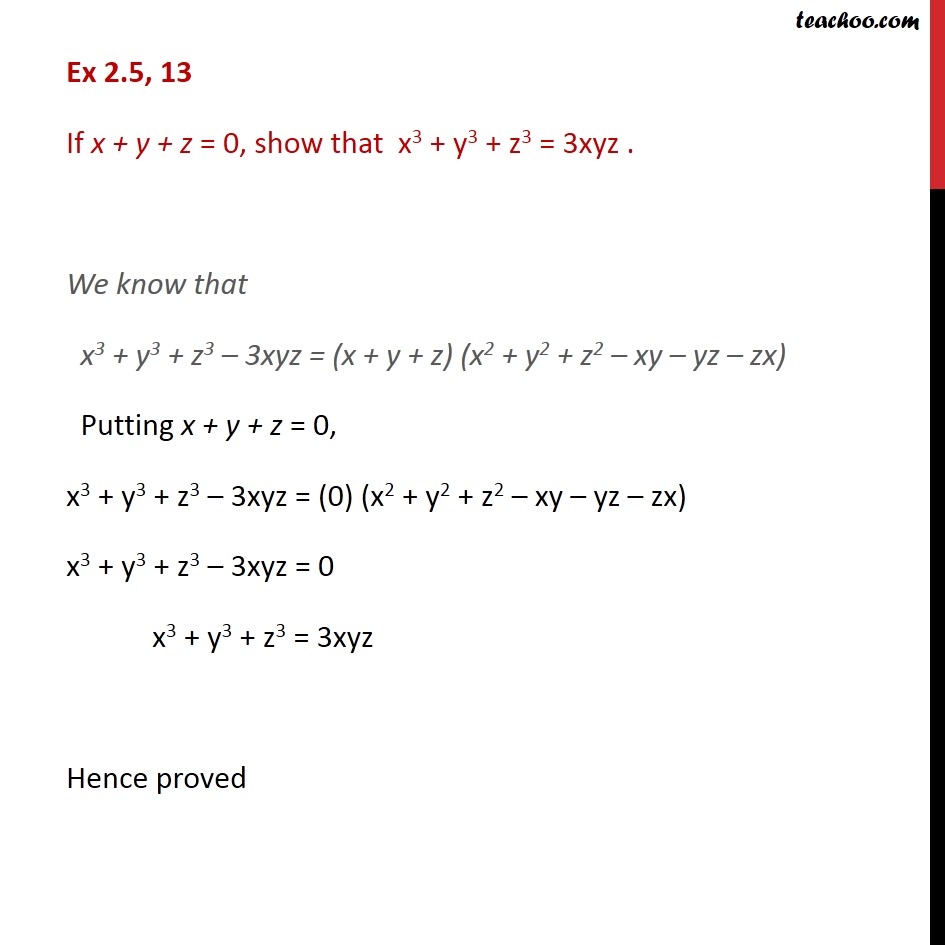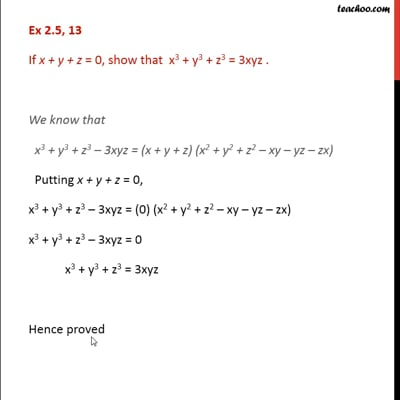Identity VIII

Chapter 2 Class 9 Polynomials (Term 2)
Concept wiseThis video is only available for Teachoo black users

### Transcript

Ex 2.5, 13 If x + y + z = 0, show that x3 + y3 + z3 = 3xyz . We know that x3 + y3 + z3 3xyz = (x + y + z) (x2 + y2 + z2 xy yz zx) Putting x + y + z = 0, x3 + y3 + z3 3xyz = (0) (x2 + y2 + z2 xy yz zx) x3 + y3 + z3 3xyz = 0 x3 + y3 + z3 = 3xyz Hence proved